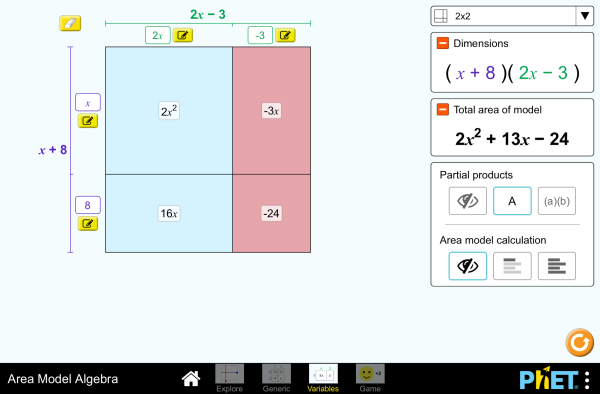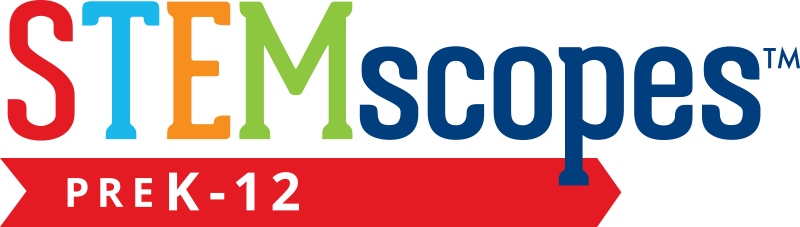# Area Model Algebra

Ova simulacija nije prevedena na ovaj jezik. I dalje ispod možete pristupiti engleskoj verziji.PreuzimanjeUgrađivanje zatvorite Ugradite interaktivnu verziju ove simulacije Koristite ovaj HTML kod da ugradite interaktivnu verziju ove simulacije. Možete promijeniti širinu i visinu ugrađene simulacije tako što ćete doraditi "width"(širina) i "height" (visina) atribute u HTML kodu. Ugradite sliku koja će na klik pokrenuti simulaciju
Kliknite za pokretanje
Koristite ovaj HTML kod da biste prikazali sliku ekrana sa riječima "Kliknite za pokretanje". Polinomi Čimbenici Produkti PhET je podržan odi učitelja poput Vas.

• Polinomi
• Čimbenici
• Produkti

### Opis

Build rectangles of various sizes and relate multiplication to area. Discover new strategies for multiplying algebraic expressions. Use the game screen to test your multiplication and factoring skills!

### Uzorak ciljeva učenja

• Develop and justify a method to use the area model to determine the product of a monomial and a binomial or the product of two binomials.
• Factor an expression, including expressions containing a variable.
• Recognize that area represents the product of two numbers and is additive.
• Represent a multiplication problem as the area of a rectangle, proportionally or using generic area.
• Develop and justify a strategy to determine the product of two multi-digit numbers by representing the product as an area or the sum of areas.

### Usklađivanje standarda

#### Zajednička jezgra - Matematika

3.MD.C.7
Relate area to the operations of multiplication and addition.
3.MD.C.7c
Use tiling to show in a concrete case that the area of a rectangle with whole-number side lengths a and b + c is the sum of a × b and a × c. Use area models to represent the distributive property in mathematical reasoning.
3.MD.C.7d
Recognize area as additive. Find areas of rectilinear figures by decomposing them into non-overlapping rectangles and adding the areas of the non-overlapping parts, applying this technique to solve real world problems.
4.NBT.B.5
Multiply a whole number of up to four digits by a one-digit whole number, and multiply two two-digit numbers, using strategies based on place value and the properties of operations. Illustrate and explain the calculation by using equations, rectangular arrays, and/or area models.
6.EE.A.3
Apply the properties of operations to generate equivalent expressions. For example, apply the distributive property to the expression 3 (2 + x) to produce the equivalent expression 6 + 3x; apply the distributive property to the expression 24x + 18y to produce the equivalent expression 6 (4x + 3y); apply properties of operations to y + y + y to produce the equivalent expression 3y.
6.EE.A.4
Identify when two expressions are equivalent (i.e., when the two expressions name the same number regardless of which value is substituted into them). For example, the expressions y + y + y and 3y are equivalent because they name the same number regardless of which number y stands for..
6.NS.B.4
Find the greatest common factor of two whole numbers less than or equal to 100 and the least common multiple of two whole numbers less than or equal to 12. Use the distributive property to express a sum of two whole numbers 1-100 with a common factor as a multiple of a sum of two whole numbers with no common factor. For example, express 36 + 8 as 4 (9 + 2)..
7.EE.A.1
Apply properties of operations as strategies to add, subtract, factor, and expand linear expressions with rational coefficients.
7.NS.A.2c
Apply properties of operations as strategies to multiply and divide rational numbers.
8.EE.C.7b
Solve linear equations with rational number coefficients, including equations whose solutions require expanding expressions using the distributive property and collecting like terms.
Verzija 1.2.1

### Savjeti učiteljaPregled kontrole simulacije, pojednostavljenje modela i uvid u učenička razmišljanja ( PDF ).

### Video (Primer)

Molimo prijavite se da biste gledali video (primer)

### Aktivnosti predane od strane učitelja

Expanding and Factoring Algebraic ExpressionsAmanda McGarry MS Guided Mathematics
Algebraic ExpressionsAmanda McGarry MS Guided Mathematics
Exploring Factoring as Undoing the Distributive PropertySarah Hampton HS
MS
Guided Mathematics
Middle School Math Sim AlignmentAmanda McGarry MS Other Mathematics
Using the area model with expressionsJennifer Knudsen, Teresa Lara-Meloy, Amanda McGarry MS Discuss Mathematics
Would You Rather ... Karen Gann HS Guided Mathematics
Area Model Algebra - Multiplying Polynomials Carolyn Felknor MS Guided Mathematics
ARTICULANDO ÁLGEBRA E GEOMETRIA: O CASO DAS EQUAÇÕES ALGÉBRICAS NO ESTUDO DE ÁREAS Verusca Batista Alves; Ana Carolina Costa Pereira MS HW
Lab
Mathematics
Distributive Property Review Tim Kissinger and Larry Peters MS Discuss
Guided
Mathematics
Jezik Preuzmite ili pokrenite Savjeti
Arapski Sve العربيةجبر نموذج المساحة
Basque Sve EuskaraAzalera-modeloan algebra
Bosanski Sve BosanskiMatematički model površina - Algebra
Danski Sve DanskArealmodel, algebra
Finski Sve suomiPinta-ala ja algebra
Francuski Sve françaisModélisation par les aires - Algèbre
Grčki Sve ΕλληνικάΥπολογισμός εμβαδού
Gujarati Sve Gujaratiબીજગણિતનું ક્ષેત્રફળ મોડેલ -નિકુંજ સવાણી
Hindi Sve हिंदीबीजगणित -नमूने का क्षेत्रफल
Japanski Sve 日本語面積と式の展開
Kazakh Sve KazakhАуданды есептеу
Kineski (pojednostavljen) Sve 中文 (中国)面积模型代数
Kineski (traditionalni) Sve 中文 (台灣)Area Model Algebra_面積模型與代數
Korejski Sve 한국어면적 모형: 대수
Letonski Sve LatviešuLaukuma modeļa algebra
Mađarski Sve magyarTerületmodell: algebra
Mongolian Sve Монгол (Монгол)Талбайн загварын Алгебр
Nizozemski Sve NederlandsAlgebra van oppervlaktemodel
Njemački Sve DeutschFlächenmodell Algebra
Persian Sve فارسیجبر مدل مساحت
Poljski Sve polskiModel pola - wyrażenia algebraiczne
Portugalski Sve portuguêsModelo de Área: Álgebra
Portugalski (Brazil) Sve português (Brasil)Modelo de Área: Álgebra
Ruski Sve русскийВычисление площади
Srpski Sve СрпскиМатематика преко површина - Алгебра
Španjolski Sve españolModelo de Áreas: ÁlgebraŠpanjolski (Mexico) Sve español (México)Modelo de Áreas: ÁlgebraŠpanjolski (Peru) Sve español (Perú)Modelo de Área con Álgebra
Švedski Sve svenskaAreamodellen och algebra
Talijanski Sve italianoRappresentazione grafica della moltiplicazione in algebra
Tamil Sve Tamilமாதிரி உருவின் எண்(அட்சர) கணிதம்
Turski Sve TürkçeAlan Modeli İle Cebir
Ukrajinski Sve українськаПлощинна модель. АлгебраVijetnamski Sve Tiếng ViệtĐại số với mô hình diện tích

The new HTML5 sims can run on iPads and Chromebooks, as well as PC, Mac, and Linux systems.

iOS 12+ Safari

Android:
Not officially supported. If you are using the HTML5 sims on Android, we recommend using the latest version of Google Chrome.

Chromebook:
The HTML5 and Flash PhET sims are supported on all Chromebooks.
Chromebook compatible sims

Windows Systems:

Macintosh Systems:

Linux Systems:

Dizajnerski tim Biblioteke treće strane Zahvale
• Jonathan Olson (developer)
• Karina Hensberry
• Susan Miller
• Ariel Paul
• Kathy Perkins
• Mariah Hermsmeyer (artwork)
• Diana López Tavares (artwork)
• almond-0.2.9.js
• base64-js-1.2.0.js
• FileSaver-b8054a2.js
• font-awesome-4.5.0
• game-up-camera-1.0.0.js
• he-1.1.1.js
• himalaya-0.2.7.js
• jama-1.0.2
• jquery-2.1.0.js
• lodash-4.17.4.js
• pegjs-0.7.0.js
• seedrandom-2.4.2.js
• text-2.0.12.js
• TextEncoderLite-3c9f6f0.js